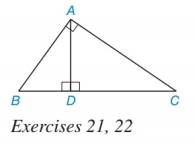Chapter 5.1, Problem 22EElementary Geometry For College St...

7th Edition
Alexander + 2 others
ISBN: 9781337614085

Solutions

Chapter
SectionElementary Geometry For College St...

7th Edition
Alexander + 2 others
ISBN: 9781337614085
Textbook Problem

In Exercises 17 to 22, use proportions to solve each problem.Assume that AB is the geometric mean of BD and BC. a) Find AB if BD = 6 and DC =10. b) Find DC if AB =106 and BC = 15.To determine

a)

To Find:

The value of AB.

Explanation

If b is the geometric mean of a and c, then b2=ac.

Calculation:

It is given that AB is the geometric mean of BD and BC.

Use the concept of geometric mean.

AB2=BDBC

Now, BC = BD + DC

Substitute BD = 6 and DC = 10 in BC = BD + DC.

BC = 6 + 10

= 16

Substitute BD = 6 and BC = 16 in AB2=BDBC

To determine

b)

To Find:

The value of DC.

Still sussing out bartleby?

Check out a sample textbook solution.

See a sample solution

The Solution to Your Study Problems

Bartleby provides explanations to thousands of textbook problems written by our experts, many with advanced degrees!

Get Started

In Exercises 516, evaluate the given quantity. log1,000

Finite Mathematics and Applied Calculus (MindTap Course List)

In Problems 27-32, find the general solution to each differential equation.

Mathematical Applications for the Management, Life, and Social Sciences

Let f(x) = 1x+1 and g(x) = x2 + 1. Find the rules for (a) f + g, (b) fg (c) f t, and (d) g f.

Applied Calculus for the Managerial, Life, and Social Sciences: A Brief Approach

Find the limit. limx1(1x1+1x23x+2)

Calculus: Early Transcendentals

True or False: is a convergent series.

Study Guide for Stewart's Multivariable Calculus, 8th

0 1 −∞ does not exist

Study Guide for Stewart's Single Variable Calculus: Early Transcendentals, 8th